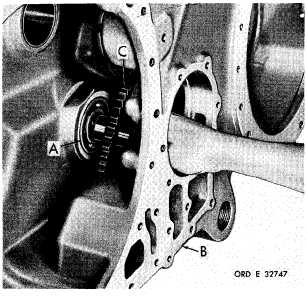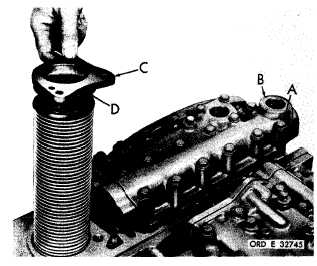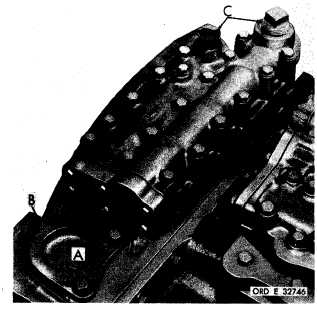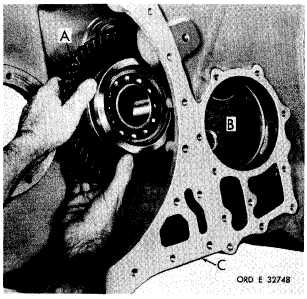Custom SearchP A R   2 2 7 - 2 2 8 A S S E M B LY C H A P   5,   SEC  X X X I II Figure   326   (Step   87) Install   eighteen   5/16-18   x   3-1/4   bolts   (A)   with flat   washers   to   retain   lockup   shift   and   pres- sure   regulator   valve   body   assembly   (B).   Us- ing   a   1/2-inch   wrench,   torque   bolts   to   13-16 p o u n d - f e e t .    I n s t a l l    m a i n    o i l    s c r e e n    a s s e m b l y (C).   Be   sure   that   oil   seal   ring   (D)   is   in   place. Figure   327   (Step   88) Install   three   3/8-24   x   1-1/8   bolts   (A)   with   lock w a s h e r s    t o    r e t a i n    m a i n    o i l    s c r e e n    a s s e m b ly ( B ) .    U s i n g    a    9 / 1 6 - i n c h    w r e n c h ,    t o r q u e    b o l ts t o    3 3 - 4 0    p o u n d - f e e t .    F o r    c l e a n l i n e s s ,    t e m p o- rarily   install   two   1-inch   pipe   plugs   (C). 2 2 8 .    A S S E M B L Y    S T E P S   – I N P U T    T R A N S F E R    A S S E M B LY Figure   328   (Step   1) Install   bearing   assembly   (A)   in   input   transfer housing (B). Install input oil pump drive gear   (C). Figure   329   (Step   2) I n s t a l l    i n p u t    t r a n s f e r    i d l e r    c l u s t e r    g e a r    a nd bearing   assembly   (A),   alining   it   with   spindle bore   (B)   in   housing   (C). 1 8 5